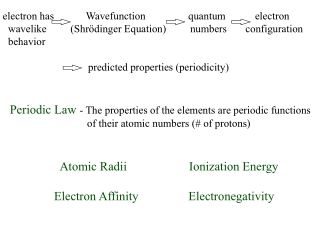DownloadDownload Presentationelectron has Wavefunction quantum electron

# electron has Wavefunction quantum electron

Download Presentation## electron has Wavefunction quantum electron

- - - - - - - - - - - - - - - - - - - - - - - - - - - E N D - - - - - - - - - - - - - - - - - - - - - - - - - - -
##### Presentation Transcript

1. electron has Wavefunction quantum electron wavelike (Shrödinger Equation) numbers configuration behavior predicted properties (periodicity) Periodic Law - The properties of the elements are periodic functions of their atomic numbers (# of protons) Atomic Radii Ionization Energy Electron Affinity Electronegativity

2. Cl Cl Atomic Radii Effective Nuclear Charge - Nuclear charge experienced by the outer shell electrons 2r The inner shell electrons shield the outer shell electrons from the full effect of the nuclear charge. Increase Increase Increase

3. Ionic Radii Neutral Atom  Anion  size Neutral Atom  Cation  size

4. Isoelectronic - species that have the same number of electrons The species with the most protons will have the smallest radius

5. First Ionization Energy - The minimum amount of energy required to remove the most loosely bound electron from an isolated gaseous atom to form an ion with a 1+ charge X(g) + 1st Ionization Energy  X+(g) + e-

6. First Ionization Energy Trend Increase Increase Increase

7. Electron Affinity - the amount of energy absorbed when an electron is added to an isolated gaseous atom to form an ion with a 1- charge. X(g) + e-(g) X-(g) Increases = less negative or more positive Increase Increase Increase Book pg 247

8. Electronegativity - measure of the relative tendency of an atom to attract electrons to itself when it is chemically combined with another atom. (qualitative) Increase Increase Increase

9. Reactions of Hydrogen Displacement Reactions: 3Fe(s) + 4H2O  Fe3O4(s) + 4H2(g) Zn(s) + 2HCl(aq) ZnCl2(s) + 4H2(g) Electrolysis: 2H 2O(g) 2H2(g) + O2(g) Combustion: 2H2(g) + O2(g)2H 2O(g) + Energy Electricity Spark or heat

10. Preparation & Rxn’s of Hydrides (Binary H containing compound) Ionic hydrides - MH M=group IA MH2 M=heavier group IIA (Ca, Sr, Ba) 2Li(l) + H2(g) 2LiH(s) Ca(l) + H2(g) CaH2(s) LiH(s) + H2O  Li(OH)(s) + H2(g) CaH2(s) + 2H2O  Ca(OH)2(s) + 2H2(g) Molecular hydrides - X2 + H2(g)HX(g) X=halogen (F,Cl,Br,I) (acidic) Combination: Ionic hydrides are basic in water Combination: Figure 6-4 (pg. 253) H2O, H2S, H2Se, H2Te

11. Oxygen Oxides - Binary compounds that contain oxygen Normal oxides - (O2-) ox # O = -2 Peroxides - (O22-) ox # O = -1 Superoxides - (O2-) ox # O = -(1/2) Basicity Increases Rxn of oxygen with metals: 4Li(s) + O2(g) (excess)  2Li2O(s) 2Na(s) + O2(g) (excess) Na2O2(g) M(s) + O2(g) (excess) MO2(s) M=K, Rb & Cs M(s) + O2(g) (excess)  MO(s) M=Be, Mg, Ca, Sr Ba(s) + O2(g) (excess)BaO2(s) pg. 257

12. Rxn of oxygen with metals (cont.): 2Fe(s) + O2(g) FeO(s) iron (II) oxide (ferrous oxide) 6FeO(s) + O2(g) Fe3O4(s) magnetic iron oxide (mixed oxide) 4Fe3O4(s) + O2(g) Fe2O3(s) iron (III) oxide (feric oxide) Rxn of oxygen with nonmetals: 2C(s) (excess) + O2(g)2CO(s) ox # C in CO = +2 C(s) + O2(g) (excess) CO2(g) ox # C in CO2 = +4

13. Rxn of non-metal oxides (acid anhydrides or acidic oxides) CO2(g) + H2O2H2CO3(aq) Acidity Increases +4 +4 Ternary Acid non-metal oxides combine with water to form ternary acids without a change in oxidation state of the non-metal Rxn of metal oxides with non-metal oxides: CaO(s) + SO3(l) CaSO4(s) metal oxides combine with non-metal oxides to produce salts without a change in oxidation states.

14. Combustion Reactions - combination reaction with oxygen. Combustion of fossil fuels: 2C8H18(l) + 25O2(g)(excess)  16CO2(g) + 18H2O(l) 2C8H18(l) + 17O2(g) 16CO(g) + 18H2O(l) 2C8H18(l) + 9O2(g)(excess)  16C(s) + 18H2O(l) S8(s) + 8O2(g) 8SO2(g) 2SO2(g) + O2(g) 2SO3(l) SO3(l) + H2O  H2SO4(l) N2(g) + O2(g)NO(g) 2NO(g) + O2(g) 2NO2(g) 3NO2(g) + H2O  HNO3(aq) + NO(g) slow reddish-brown gas UV light Acid Rain Acid Rain